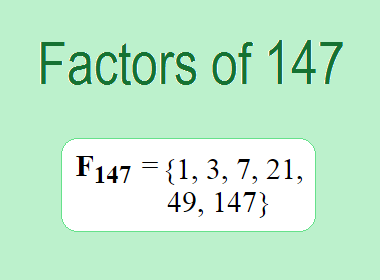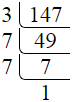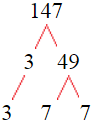# Factors of 147The factors of 147 are 1, 3, 7, 21, 49, and 147 i.e. F147 = {1, 3, 7, 21, 49, 147}. The factors of 147 are all the numbers that can divide 147 without leaving a remainder.

We can check if these numbers are factors of 147 by dividing 147 by each of them. If the result is a whole number, then the number is a factor of 147. Let's do this for each of the numbers listed above:

·        1 is a factor of 147 because 147 divided by 1 is 147.

·        3 is a factor of 147 because 147 divided by 3 is 49.

·        7 is a factor of 147 because 147 divided by 7 is 21.

·        21 is a factor of 147 because 147 divided by 21 is 7.

·        49 is a factor of 147 because 147 divided by 49 is 3.

·        147 is a factor of 147 because 147 divided by 147 is 1.

## How to Find Factors of 147?

1 and the number itself are the factors of every number. So, 1 and 147 are two factors of 147. To find the other factors of 147, we can start by dividing 147 by the numbers between 1 and 147. If we divide 147 by 2, we get a remainder of 1. Therefore, 2 is not a factor of 147. If we divide 147 by 3, we get a remainder of 0. Therefore, 3 is a factor of 147.

Next, we can check if 4 is a factor of 147. If we divide 147 by 4, we get a remainder of 3. Therefore, 4 is not a factor of 147. We can continue this process for all the possible factors of 147.

Through this process, we can find that the factors of 147 are 1, 3, 7, 21, 49, and 147. These are the only numbers that can divide 147 without leaving a remainder.

********************

********************

## Properties of the Factors of 147

The factors of 147 have some interesting properties. One of the properties is that the sum of the factors of 147 is equal to 228. We can see this by adding all the factors of 147 together:

1 + 3 + 7 + 21 + 49 + 147 = 228

Another property of the factors of 147 is that they are all odd numbers. This is because 147 is an odd number, and any even number cannot divide an odd number without leaving a remainder.

Another property of the factors of 147 is that the prime factors of 147 are 3 and 7 only.

## Applications of the Factors of 147

The factors of 147 have several applications in mathematics. One of the applications is in finding the highest common factor (HCF) of two or more numbers. The HCF is the largest factor that two or more numbers have in common. For example, to find the HCF of 147 and 42, we need to find the factors of both numbers and identify the largest factor they have in common. The factors of 147 are 1, 3, 7, 21, 49, and 147. The factors of 42 are 1, 2, 3, 6, 7, 14, 21, and 42. The largest factor that they have in common is 21. Therefore, the HCF of 147 and 42 is 21.

Another application of the factors of 147 is in prime factorization. Prime factorization is the process of expressing a number as the product of its prime factors. The prime factors of 147 are 3 and 7, since these are the only prime numbers that can divide 147 without leaving a remainder. Therefore, we can express 147 as:

147 = 3 × 7 × 7

We can do prime factorization by division and factor tree method also. Here is the prime factorization of 147 by division method,147 = 3 × 7 × 7

Here is the prime factorization of 147 by the factor tree method,147 = 3 × 7 × 7

## Conclusion

The factors of 147 are the numbers that can divide 147 without leaving a remainder. The factors of 147 are 1, 3, 7, 21, 49, and 147. The factors of 147 have some interesting properties, such as being odd numbers and having a sum of 228. The factors of 147 have several applications in mathematics, such as finding the highest common factor and prime factorization.